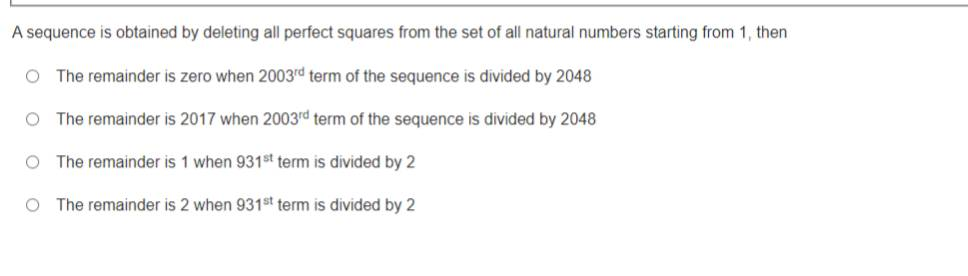# A sequence is obtained by deleting all perfect squares from the set of all natural numbers starting from 1, then

69 views

recategorized

A sequence is obtained by deleting all perfect squares from the set of all natural numbers starting from 1, thenby (45.9k points)

Correct option is (C) The reminder is 1 when 931th term is divided by 2

Given sequence is

2,3,5,7,8,10,11,12,13,14,15,17,18,19,20,21,22,23,24,26......

2003rd term = ?

1892th term = 1937

1980th term = 2026

2003rd term = 2026 + (2003 - 1980)

= 2026 + 23 = 2049

870th term = 901

930th term = 962

$\therefore$ 931st term = 963

(a, b) $\frac{T_{2003}}{2048}=\frac{2049}{2048}$ the reminder left 1

option (a), and (b) are false

(c, d) $\frac{T_{931}}2=481+\frac12$

Thus, reminder left 1

option (c) is correct